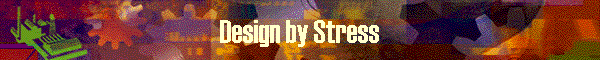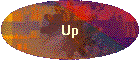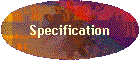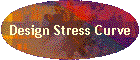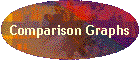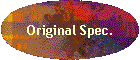This function allows users to define stress curve and reverse calculate dimension data from the defined stress data. To define a target stress curve, DynaRod developed a sub tool, Stress Curve Drawer which works on Excel workbook.  Stress Curve Drawer is invoked from DynaRod and returns the defined stress data for the calculation of dimension.  The defined stress curve must be on dynamic calculation basis since the reverse calculation logics for dimension uses dynamic type calculation.  To utilize this function as desired, user may need to know well about the shape of stress curve which is calculated by dynamic logics. If you define the target stress curve which is based on Garrison' math image here, the resulted dimension may not be as you expected. To design a completely new taper As this program is so designed to start with a template rod in order to prepare the starting dimensions of a rod, user must select an existing rod as a template. Then how to design a rod taper "from scratch"? It is WHEN changing the target Stress Curve on "Stress Curve Drawer" tool.   Just neglect the existing Stress Curve of template and newly define the Stress Curve line on the tool.   [Design by Stress] program in DynaRod receives the newly defined Stress Curve and calculate the newly designed dimensions as a completely new taper.